A high-energy electron deflected from a straight-line trajectory emits electromagnetic radiation that is referred to as “synchrotron radiation.” The radiation is highly collimated in the direction of propagation of the electron but is very broadband (Figure S1). A key trick to narrowing the bandwidth is to subject the electron to a periodic path where interference effects enhance a narrow spectrum but cancel most other wavelengths (Figure S2). The more periods to this periodic motion, the narrower the radiation spectrum. This is referred to as “undulator radiation,” which commonly is accomplished by directing the electron beam through a periodic arrangement of strong magnets, referred to as an “undulator.”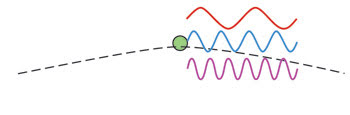Figure S1. Broadband synchrotron radiation (represented here by three waves of different wavelengths) is emitted by a high-energy electron deflected from a straight-line trajectory (represented here by the broken line).

When a collection of electrons is directed through an undulator, the observed radiation is a linear superposition of the radiated fields from the individual electrons. Thus, if all the electrons are contained within a bunch that is much smaller than the undulator radiation wavelength, their fields add in phase, resulting in “coherent undulator radiation.”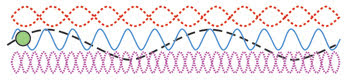Figure S2. Forcing the electron to follow a periodic path (the black broken line) causes most of the synchrotron-radiation wavelengths to interfere destructively (the orange and violet waves), but a narrow band of wavelengths interferes constructively (the blue wave).

For the x-ray wavelengths of interest to us, however, the electron bunches entering the undulator are much bigger than the emitted wavelength, and the net radiated field is the result of the uneven charge distribution within the bunch (Figure S3). This initially weak undulator radiation field interacts with the electrons inside the bunch, accelerating or decelerating them, depending on their location within the bunch.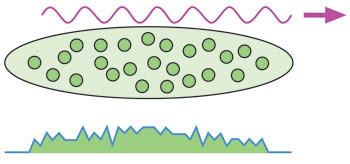Figure S3.
The uneven distribution of electrons in a bunch results in a weak, but nonzero, undulator radiation field.

This results in an electron density modulation that has the same wavelength as the undulator radiation and that consequently can enhance the initially weak undulator radiation amplitude. This, in turn, increases the internal density modulation within the bunch (Figure S4).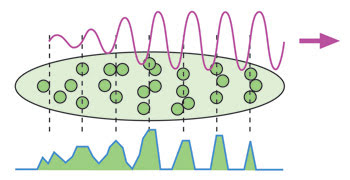Figure S4. The initially weak undulator radiation field accelerates some electrons and decelerates others, creating an electron-density modulation with the same period as the undulator radiation.

Thus, similar to the amplification mechanism in an energized atomic medium, a gain mechanism for the undulator radiation is produced. This process is referred to as “Self-Amplified Spontaneous Emission” (SASE).

Photonics Spectra
Jul 2008
x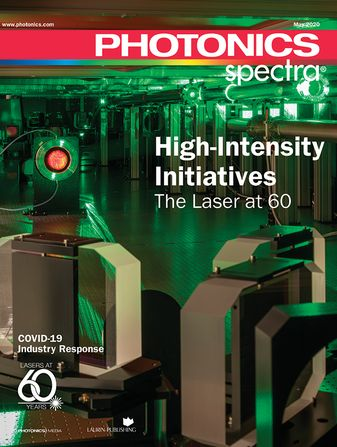Subscribe to Photonics Spectra magazine - FREE!